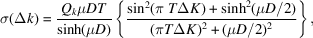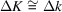International
Tables for
Crystallography
Volume C
Mathematical, physical and chemical tables
Edited by E. Prince

International Tables for Crystallography (2006). Vol. C, ch. 6.4, p. 612

## Section 6.4.14. Relationship with the dynamical theory

T. M. Sabinea

aANSTO, Private Mail Bag 1, Menai, NSW 2234, Australia

### 6.4.14. Relationship with the dynamical theory

| top | pdf |

Sabine & Blair have shown that the two classical limits for the integrated intensity in the symmetric Bragg case can be obtained from the Hamilton–Darwin equations when the dynamic refractive index of the crystal is explicitly taken into account. Their treatment is based on the following expression for σ(Δk):where ΔK refers to the scattering vector within the crystal. Use of the relationand the replacement of the Fresnellian by a Lorentzian leads to equation (6.4.5.1)with the inclusion of C (6.4.5.2). The relationship between ΔK and Δk, which is a function of the dynamic refractive index of the crystal, is derived in the original publication. Insertion of this expression into equations (6.4.4.3)and (6.4.4.4)and integration over Δk, since the diffracted beam is observed outside the crystal, leads to a dynamic extinction factor, which can be compared with the values determined from the equations given in Section 6.4.5. The integrations cannot be carried out analytically and require numerical calculation in each case.

Olekhnovich & Olekhnovich (1978, 1980) have given limited expressions for primary extinction in the parallelepiped and the cylinder based on the equations of the dynamical theory in the non-absorbing case. Comparisons with the results of the present theory are given by Sabine (1988) and Sabine, Von Dreele & Jørgensen (1988).

### References

Olekhnovich, N. M. & Olekhnovich, A. I. (1978). Primary extinction for finite crystals. Square-section parallelepiped. Acta Cryst. A34, 321–326.
Olekhnovich, N. M. & Olekhnovich, A. I. (1980). Primary extinction for finite crystals. Cylinder. Acta Cryst. A36, 22–27.
Sabine, T. M. (1988). A reconciliation of extinction theories. Acta Cryst. A44, 368–373.
Sabine, T. M., Von Dreele, R. B. & Jørgensen, J.-E. (1988). Extinction in time-of-flight neutron powder diffractometry. Acta Cryst. A44, 374–379.